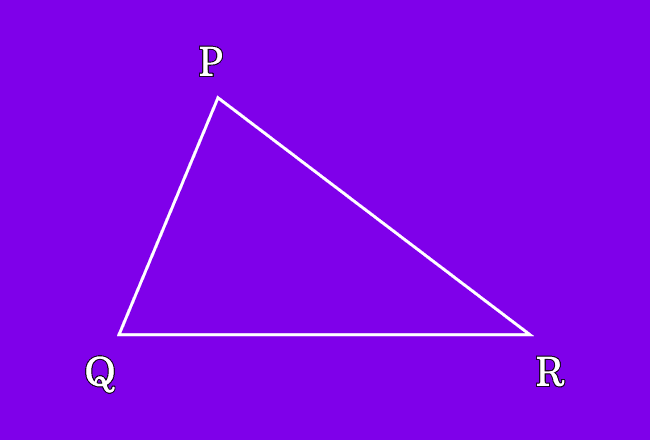# Vertices of a Triangle

A point where any two sides of a triangle meet, is called a vertex of a triangle.

## Introduction

Geometrically, a triangle is formed by the intersection of three line segments. Each side of a triangle has two endpoints and the endpoints of all three sides are intersected possibly at three different points in a plane for forming a triangle. The three different intersecting points of them are called vertices of a triangle.

### Example

$\Delta PQR$ is a triangle and it is formed by three line segments.1. The sides $\small \overline{PQ}$ and $\small \overline{PR}$ are intersected at point $\small P$. So, the point $\small P$ is called as a vertex.
2. The sides $\small \overline{PQ}$ and $\small \overline{QR}$ are intersected at point $\small Q$. So, the point $\small Q$ is called as a vertex.
3. The sides $\small \overline{PR}$ and $\small \overline{QR}$ are intersected at point $\small R$. So, the point $\small R$ is called as a vertex.

Therefore, the intersecting points $P$, $Q$ and $R$ are called the vertices of the triangle $PQR$.

Latest Math Topics
Jun 26, 2023
Jun 23, 2023

Latest Math Problems
Jul 01, 2023
Jun 25, 2023
###### Math Questions

The math problems with solutions to learn how to solve a problem.

Learn solutions

Practice now

###### Math Videos

The math videos tutorials with visual graphics to learn every concept.

Watch now

###### Subscribe us

Get the latest math updates from the Math Doubts by subscribing us.